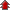Home Board Paper Solutions Text Book Solutions Articles NCERT Exemplar Solutions

# Alternating Current### Exercise 1

•  Q1 A 100 Ω resistor is connected to a 220 V, 50 Hz ac supply. (a) What is the rms value of current in the circuit? (b) What is the net power consumed over a full cycle? Q2 (a) The peak voltage of an ac supply is 300 V. What is the rms voltage? (b) The rms value of current in an ac circuit is 10 A. What is the peak current? Q3 A 44 mH inductor is connected to 220 V, 50 Hz ac supply. Determine the rms value of the current in the circuit.
• NCERT Chapter